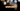# Leetcode | Solution of Search Insert Position in JavaScript

March 28th, 2020
|

In this post, we will solve search insert position from leetcode and compute it's time and space complexities. Let's begin.

# Problem Statement

The question can be found at leetcode search insert position problem.

The problem states that we are given a sorted array in ascending order and a target number. We need to find a position in which if the number is placed, it'll still be sorted.

# Constraints and challenges

The following constraints are given in the question

• The array has no duplicates

We'll see a solution, which will work even if the array had duplicates.

# Solution

We will discuss a solution using simple for loop

We'll take the following approach

• We'll search for a number that is greater than or equal to the target.
• The position where the number is found is the position at which we need to place the target

For example, if the array is `[1,3,5,7]` and the target is `6`. We'll search for a number greater than or equal to `6`. The answer is `7` and its position is `3`. So the answer is 3.

We have discussed the approach, I urge you to go ahead on leetcode and give it another try.If you are here, it means something went wrong in implementation or you are just too lazy. In any case, let's see a simple implementation of the above logic.
``````var searchInsert = function (nums, target) {

for (let index = 0; index < nums.length; index++) {
if (target <= nums[index]) {
return index;
}
}
return nums.length;
};``````

Let's look at the solution. We have a loop where we look for the number greater than or equal to the target. When found, we return that position.

At the last, we are returning the length of the nums array. It is to handle two very important edge cases.

• Array length is 0. In this case, we'll need to put the number at the first position (zero-index)
• The number is greater than all the elements in the array. Then we'll need to put at last i.e. array length index

Here are the stats

``````Status: Accepted
Runtime: 60ms
Memory: 34.7MB``````

## Time and space complexity

### Time complexity

We are looping over the number array once in the worst case, so time complexity would be O(n). We can also use the binary search here to improve the complexity to O(log n).

### Space complexity

We are not using any extra space. So space complexity is constant, O(1).

# Summary

So, we solved the search insert position problem by using a simple while loop and calculated the time and space complexities.

I hope you enjoyed solving this question. This is it for this one, complete source code for this post can be found on my Github Repo. Will see you in the next one.